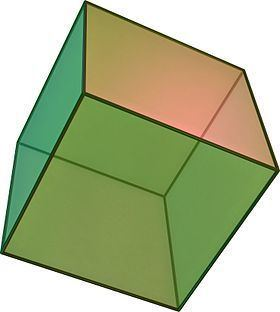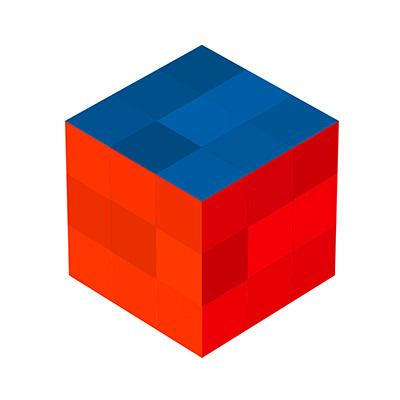# Cube

Updated on
Covid-19
 Named after  Q3Diameter  3Automorphisms  48Volume  a³Number of edges  12Number of faces  6 Radius  3Girth  4Chromatic number  2Surface area  6 × a²Number of vertices  8Base shape  Square Common objects with this shape  Rubik's Cube, Box, Dice, Ice cube

In geometry, a cube is a three-dimensional solid object bounded by six square faces, facets or sides, with three meeting at each vertex.

## Contents

The cube is the only regular hexahedron and is one of the five Platonic solids. It has 6 faces, 12 edges, and 8 vertices.

The cube is also a square parallelepiped, an equilateral cuboid and a right rhombohedron. It is a regular square prism in three orientations, and a trigonal trapezohedron in four orientations.The cube is dual to the octahedron. It has cubical or octahedral symmetry.

## Orthogonal projectionsThe cube has four special orthogonal projections, centered, on a vertex, edges, face and normal to its vertex figure. The first and third correspond to the A2 and B2 Coxeter planes.

## Spherical tiling

The cube can also be represented as a spherical tiling, and projected onto the plane via a stereographic projection. This projection is conformal, preserving angles but not areas or lengths. Straight lines on the sphere are projected as circular arcs on the plane.

## Cartesian coordinates

For a cube centered at the origin, with edges parallel to the axes and with an edge length of 2, the Cartesian coordinates of the vertices are

(±1, ±1, ±1)

while the interior consists of all points (x0, x1, x2) with −1 < xi < 1.

## Equation in R3

In analytic geometry, a cube's surface with center (x0, y0, z0) and edge length of 2a is the locus of all points (x, y, z) such that

max { | x x 0 | , | y y 0 | , | z z 0 | } = a .

## Formulas

For a cube of edge length a :

As the volume of a cube is the third power of its sides a × a × a , third powers are called cubes, by analogy with squares and second powers.

A cube has the largest volume among cuboids (rectangular boxes) with a given surface area. Also, a cube has the largest volume among cuboids with the same total linear size (length+width+height).

## Point in space

For a cube whose circumscribing sphere has radius R, and for a given point in its 3-dimensional space with distances di from the cube's eight vertices, we have:

i = 1 8 d i 4 8 + 16 R 4 9 = ( i = 1 8 d i 2 8 + 2 R 2 3 ) 2 .

## Doubling the cube

Doubling the cube, or the Delian problem, was the problem posed by ancient Greek mathematicians of using only a compass and straightedge to start with the length of the edge of a given cube and to construct the length of the edge of a cube with twice the volume of the original cube. They were unable to solve this problem, and in 1837 Pierre Wantzel proved it to be impossible because the cube root of 2 is not a constructible number.

## Uniform colorings and symmetry

The cube has three uniform colorings, named by the colors of the square faces around each vertex: 111, 112, 123.

The cube has three classes of symmetry, which can be represented by vertex-transitive coloring the faces. The highest octahedral symmetry Oh has all the faces the same color. The dihedral symmetry D4h comes from the cube being a prism, with all four sides being the same color. The lowest symmetry D2h is also a prismatic symmetry, with sides alternating colors, so there are three colors, paired by opposite sides. Each symmetry form has a different Wythoff symbol.

## Geometric relations

A cube has eleven nets (one shown above): that is, there are eleven ways to flatten a hollow cube by cutting seven edges. To color the cube so that no two adjacent faces have the same color, one would need at least three colors.

The cube is the cell of the only regular tiling of three-dimensional Euclidean space. It is also unique among the Platonic solids in having faces with an even number of sides and, consequently, it is the only member of that group that is a zonohedron (every face has point symmetry).

The cube can be cut into six identical square pyramids. If these square pyramids are then attached to the faces of a second cube, a rhombic dodecahedron is obtained (with pairs of coplanar triangles combined into rhombic faces).

## Other dimensions

The analogue of a cube in four-dimensional Euclidean space has a special name—a tesseract or hypercube. More properly, a hypercube (or n-dimensional cube or simply n-cube) is the analogue of the cube in n-dimensional Euclidean space and a tesseract is the order-4 hypercube. A hypercube is also called a measure polytope.

There are analogues of the cube in lower dimensions too: a point in dimension 0, a segment in one dimension and a square in two dimensions.

The quotient of the cube by the antipodal map yields a projective polyhedron, the hemicube.

If the original cube has edge length 1, its dual polyhedron (an octahedron) has edge length 2 / 2 .

The cube is a special case in various classes of general polyhedra:

The vertices of a cube can be grouped into two groups of four, each forming a regular tetrahedron; more generally this is referred to as a demicube. These two together form a regular compound, the stella octangula. The intersection of the two forms a regular octahedron. The symmetries of a regular tetrahedron correspond to those of a cube which map each tetrahedron to itself; the other symmetries of the cube map the two to each other.

One such regular tetrahedron has a volume of 1/3 of that of the cube. The remaining space consists of four equal irregular tetrahedra with a volume of 1/6 of that of the cube, each.

The rectified cube is the cuboctahedron. If smaller corners are cut off we get a polyhedron with six octagonal faces and eight triangular ones. In particular we can get regular octagons (truncated cube). The rhombicuboctahedron is obtained by cutting off both corners and edges to the correct amount.

A cube can be inscribed in a dodecahedron so that each vertex of the cube is a vertex of the dodecahedron and each edge is a diagonal of one of the dodecahedron's faces; taking all such cubes gives rise to the regular compound of five cubes.

If two opposite corners of a cube are truncated at the depth of the three vertices directly connected to them, an irregular octahedron is obtained. Eight of these irregular octahedra can be attached to the triangular faces of a regular octahedron to obtain the cuboctahedron.

The cube is topologically related to a series of spherical polyhedra and tilings with order-3 vertex figures.

The cuboctahedron is one of a family of uniform polyhedra related to the cube and regular octahedron.

The cube is topologically related as a part of sequence of regular tilings, extending into the hyperbolic plane: {4,p}, p=3,4,5...

With dihedral symmetry, Dih4, the cube is topologically related in a series of uniform polyhedra and tilings 4.2n.2n, extending into the hyperbolic plane:

All these figures have octahedral symmetry.

The cube is a part of a sequence of rhombic polyhedra and tilings with [n,3] Coxeter group symmetry. The cube can be seen as a rhombic hexahedron where the rhombi are squares.

The cube is a square prism:

As a trigonal trapezohedron, the cube is related to the hexagonal dihedral symmetry family.

## In uniform honeycombs and polychora

It is an element of 9 of 28 convex uniform honeycombs:

It is also an element of five four-dimensional uniform polychora:

## Cubical graph

The skeleton of the cube (the vertices and edges) form a graph, with 8 vertices, and 12 edges. It is a special case of the hypercube graph. It is one of 5 Platonic graphs, each a skeleton of its Platonic solid.

An extension is the three dimensional k-ary Hamming graph, which for k = 2 is the cube graph. Graphs of this sort occur in the theory of parallel processing in computers.

Cube Wikipedia

Topics